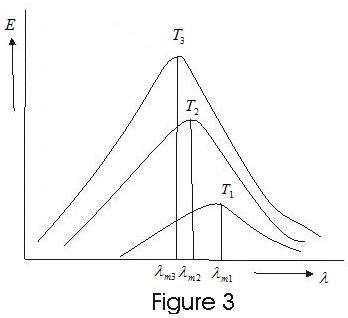# Wein's displacement law and Nature of thermal Radiation

## Wein's displacement law

• Radiation emitted by a black body is a mixture of waves of different wavelengths and only a small range of wanelength has significant countribution in the total radiation.
• A body is heated at different temperature and Enegy of radiation is plotted agains wavelength is plotted for different temperature we get following curves.• These curves show
(i) Energy is not uniformly distributed in the radiation spectreum of black body.
(ii) At a given temperature the intensity of radiations increases with increase in wavelength, becoms maximum at particular wavelength and further increase in wavelngth leads to decrease in intensity of heat radiation.
(iii) Increase in temperature causes increase in energy emission for all wavelengths.
(iv) Increase in temperature causes decrease in $\lambda _m$, where $\lambda _m$ is wavelenght corresponding to highest intersity. This wavelength $\lambda _m$ is inversily properational to the absolute temperature of the emitter.
$\lambda _m T = b$ ---(1)
Where b is a constant and this equation is known as Wein's displacement law.
$b=0.2896 \times 10^{-2}$ mk for black body and is known as Wien's constant.